Search by Topic

Resources tagged with Maths Supporting SET similar to Climbing:

Filter by: Content type:
Age range:
Challenge level:

There are 89 results

Broad Topics > Applications > Maths Supporting SETImmersion

Age 14 to 16 Challenge Level:

Various solids are lowered into a beaker of water. How does the water level rise in each case?Electric Kettle

Age 14 to 16 Challenge Level:

Explore the relationship between resistance and temperatureAir Nets

Age 7 to 18 Challenge Level:

Can you visualise whether these nets fold up into 3D shapes? Watch the videos each time to see if you were correct.Equation Matcher

Age 16 to 18 Challenge Level:

Can you match these equations to these graphs?Operating Machines

Age 16 to 18 Challenge Level:

What functions can you make using the function machines RECIPROCAL and PRODUCT and the operator machines DIFF and INT?Truth Tables and Electronic Circuits

Age 11 to 18

Investigate circuits and record your findings in this simple introduction to truth tables and logic.Maths Filler 2

Age 14 to 16 Challenge Level:

Can you draw the height-time chart as this complicated vessel fills with water?What's That Graph?

Age 14 to 16 Challenge Level:

Can you work out which processes are represented by the graphs?Ball Bearings

Age 16 to 18 Challenge Level:

If a is the radius of the axle, b the radius of each ball-bearing, and c the radius of the hub, why does the number of ball bearings n determine the ratio c/a? Find a formula for c/a in terms of n.How Do You React?

Age 14 to 16 Challenge Level:

To investigate the relationship between the distance the ruler drops and the time taken, we need to do some mathematical modelling...Taking Trigonometry Series-ly

Age 16 to 18 Challenge Level:

Look at the advanced way of viewing sin and cos through their power series.Real-life Equations

Age 16 to 18 Challenge Level:

Here are several equations from real life. Can you work out which measurements are possible from each equation?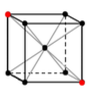Crystal Symmetry

Age 16 to 18 Challenge Level:

Use vectors and matrices to explore the symmetries of crystals.Debt Race

Age 16 to 18 Challenge Level:

Who will be the first investor to pay off their debt?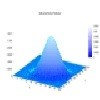Pdf Matcher

Age 16 to 18 Challenge Level:

Which pdfs match the curves?Building Approximations for Sin(x)

Age 16 to 18 Challenge Level:

Build up the concept of the Taylor seriesSpherical Triangles on Very Big Spheres

Age 16 to 18 Challenge Level:

Shows that Pythagoras for Spherical Triangles reduces to Pythagoras's Theorem in the plane when the triangles are small relative to the radius of the sphere.What Do Functions Do for Tiny X?

Age 16 to 18 Challenge Level:

Looking at small values of functions. Motivating the existence of the Taylor expansion.Whose Line Graph Is it Anyway?

Age 16 to 18 Challenge Level:

Which line graph, equations and physical processes go together?Nine Eigen

Age 16 to 18 Challenge Level:

Explore how matrices can fix vectors and vector directions.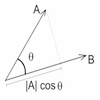Cross with the Scalar Product

Age 16 to 18 Challenge Level:

Explore the meaning of the scalar and vector cross products and see how the two are related.Fix Me or Crush Me

Age 16 to 18 Challenge Level:

Can you make matrices which will fix one lucky vector and crush another to zero?Scientific Curves

Age 16 to 18 Challenge Level:

Can you sketch these difficult curves, which have uses in mathematical modelling?Vector Walk

Age 14 to 18 Challenge Level:

Starting with two basic vector steps, which destinations can you reach on a vector walk?Polygon Walk

Age 16 to 18 Challenge Level:

Go on a vector walk and determine which points on the walk are closest to the origin.Stirling Work

Age 16 to 18 Challenge Level:

See how enormously large quantities can cancel out to give a good approximation to the factorial function.Perfect Eclipse

Age 14 to 16 Challenge Level:

Use trigonometry to determine whether solar eclipses on earth can be perfect.Transformations for 10

Age 16 to 18 Challenge Level:

Explore the properties of matrix transformations with these 10 stimulating questions.Square Pair

Age 16 to 18 Challenge Level:

Explore the shape of a square after it is transformed by the action of a matrix.Constantly Changing

Age 14 to 16 Challenge Level:

Many physical constants are only known to a certain accuracy. Explore the numerical error bounds in the mass of water and its constituents.Matrix Meaning

Age 16 to 18 Challenge Level:

Explore the meaning behind the algebra and geometry of matrices with these 10 individual problems.Construct the Solar System

Age 14 to 18 Challenge Level:

Make an accurate diagram of the solar system and explore the concept of a grand conjunction.Robot Camera

Age 14 to 16 Challenge Level:

Could nanotechnology be used to see if an artery is blocked? Or is this just science fiction?Investigating Epidemics

Age 11 to 16 Challenge Level:

Simple models which help us to investigate how epidemics grow and die out.Track Design

Age 14 to 16 Challenge Level:

Where should runners start the 200m race so that they have all run the same distance by the finish?Perspective Drawing

Age 11 to 16 Challenge Level:

Explore the properties of perspective drawing.Investigating the Dilution Series

Age 14 to 16 Challenge Level:

Which dilutions can you make using only 10ml pipettes?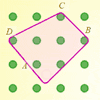Elastic Maths

Age 14 to 18

How do you write a computer program that creates the illusion of stretching elastic bands between pegs of a Geoboard? The answer contains some surprising mathematics.Age 14 to 18 Challenge Level:

How would you design the tiering of seats in a stadium so that all spectators have a good view?Witch's Hat

Age 11 to 16 Challenge Level:

What shapes should Elly cut out to make a witch's hat? How can she make a taller hat?Guessing the Graph

Age 14 to 16 Challenge Level:

Can you suggest a curve to fit some experimental data? Can you work out where the data might have come from?Pdf Stories

Age 16 to 18 Challenge Level:

Invent scenarios which would give rise to these probability density functions.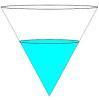Fill Me up Too

Age 14 to 16 Challenge Level:

In Fill Me Up we invited you to sketch graphs as vessels are filled with water. Can you work out the equations of the graphs?Designing Table Mats

Age 11 to 16 Challenge Level:

Formulate and investigate a simple mathematical model for the design of a table mat.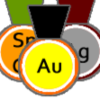Alternative Record Book

Age 14 to 18 Challenge Level:

In which Olympic event does a human travel fastest? Decide which events to include in your Alternative Record Book.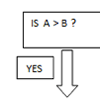Mystery Procedure

Age 14 to 16 Challenge Level:

Can you work out what this procedure is doing?Big and Small Numbers in Chemistry

Age 14 to 16 Challenge Level:

Get some practice using big and small numbers in chemistry.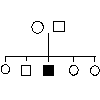Genetics

Age 14 to 16 Challenge Level:

A problem about genetics and the transmission of disease.Population Dynamics Collection

Age 16 to 18 Challenge Level:

This is our collection of tasks on the mathematical theme of 'Population Dynamics' for advanced students and those interested in mathematical modelling.Carbon Footprints

Age 14 to 16 Challenge Level:

Is it really greener to go on the bus, or to buy local?